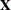# The MIXED Procedure

## Syntax: MIXED Procedure

The following statements are available in the MIXED procedure:

• PROC MIXED <options>;

• BY variables;

• CLASS variable <(REF= option)> …<variable <(REF= option)>> </ global-options>;

• CODE <options>;

• ID variables;

• MODEL dependent = <fixed-effects> </ options>;

• RANDOM random-effects </ options>;

• REPEATED <repeated-effect> </ options>;

• PARMS (value-list)…</ options>;

• PRIOR <distribution> </ options>;

• CONTRAST ’label’ <fixed-effect values …>
<|random-effect values …>, …</ options>
;

• ESTIMATE ’label’ <fixed-effect values …>
<|random-effect values …> </ options>
;

• LSMEANS fixed-effects </ options>;

• LSMESTIMATE model-effect lsmestimate-specification </ options>;

• SLICE model-effect </ options>;

• STORE <OUT=>item-store-name </ LABEL='label'>;

• WEIGHT variable;

Items within angle brackets ( < > ) are optional. The CONTRAST , ESTIMATE , LSMEANS , and RANDOM statements can appear multiple times; all other statements can appear only once.

The PROC MIXED and MODEL statements are required, and the MODEL statement must appear after the CLASS statement if a CLASS statement is included. The CONTRAST , ESTIMATE , LSMEANS , RANDOM , and REPEATED statements must follow the MODEL statement. The CONTRAST and ESTIMATE statements must also follow any RANDOM statements. The LSMESTIMATE , SLICE , and STORE statements are shared with many procedures. Summary descriptions of functionality and syntax for these statements are also given after the PROC MIXED statement in alphabetical order, but you can find full documentation on them in Chapter 19: Shared Concepts and Topics.

Table 65.1 summarizes the basic functions and important options of each PROC MIXED statement. The syntax of each statement in Table 65.1 is described in the following sections in alphabetical order after the description of the PROC MIXED statement.

Table 65.1: Summary of PROC MIXED Statements

Statement

Description

Options

Invokes the procedure

DATA= specifies input data set, METHOD= specifies estimation method

Performs multiple PROC MIXED analyses in one invocation

None

Declares qualitative variables that create indicator variables in design matrices

None

Requests that the procedure write SAS DATA step code to a file or catalog entry

FILE= names the file where the generated code is saved, CATALOG= names the catalog entry where the generated code is saved, IMPUTE imputes predicted values for observations with missing or invalid covariates, RESIDUAL computes residuals

Lists additional variables to be included in predicted values tables

None

Specifies dependent variable and fixed effects, setting upS requests solution for fixed-effects parameters, DDFM= specifies denominator degrees of freedom method, OUTP= outputs predicted values to a data set, INFLUENCE computes influence diagnostics

Specifies random effects, setting upandSUBJECT= creates block-diagonality, TYPE= specifies covariance structure, S requests solution for random-effects parameters, G displays estimatedSets upSUBJECT= creates block-diagonality, TYPE= specifies covariance structure, R displays estimated blocks of, GROUP= enables between-subject heterogeneity, LOCAL adds a diagonal matrix toSpecifies a grid of initial values for the covariance parameters

HOLD= and NOITER hold the covariance parameters or their ratios constant, PARMSDATA= reads the initial values from a SAS data set

Performs a sampling-based Bayesian analysis for variance component models

NSAMPLE= specifies the sample size, SEED= specifies the starting seed

Constructs custom hypothesis tests

E displays thematrix coefficients

Constructs custom scalar estimates

CL produces confidence limits

Computes least squares means for classification fixed effects

DIFF computes differences of the least squares means, ADJUST= performs multiple comparisons adjustments, AT changes covariates, OM changes weighting, CL produces confidence limits, SLICE= tests simple effects

Provides custom hypothesis tests among the least squares means

ADJUST= determines the method for multiple comparison adjustment of LS-mean differences, JOINT requests a joint F or chi-square test for the rows of the estimate

Performs a partitioned analysis of LS–means for an interaction

ADJUST= determines the method for multiple comparison adjustment of LS-mean differences, DIFF requests differences of LS-means

Saves the context and results of the analysis

LABEL= adds a custom label

Specifies a variable by which to weightNone